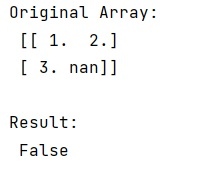# Detect if a NumPy array contains at least one non numeric value

Learn, how to detect if a NumPy array contains at least one non numeric value in Python?
Submitted by Pranit Sharma, on December 24, 2022

NumPy is an abbreviated form of Numerical Python. It is used for different types of scientific operations in python. Numpy is a vast library in python which is used for almost every kind of scientific or mathematical operation. It is itself an array which is a collection of various methods and functions for processing the arrays.

## Detecting if a NumPy array contains at least one non numeric value

While creating a DataFrame or importing a CSV file, there could be some NaN values in the cells. NaN values mean "Not a Number" which generally means that there are some missing values in the cell. Hence, we need to find the index of fulfilled values.

For this purpose, we can use numpy.isfinite() which is used to check if there is an array containing nan values or infinite values or negative infinite values. It returns True if it contains none otherwise it returns False. A better solution is to return True immediately when NAN is found.

Let us understand with the help of an example,

## Python code to detect if a NumPy array contains at least one non numeric value

```# Import numpy
import numpy as np

# Creating a numpy array
arr = np.array([[1,2],[3,np.nan]])

# Display original array
print("Original Array:\n",arr,"\n")

# Using infinite method to check
res = np.isfinite(arr).all()

# Display result
print("Result:\n",res)
```

Output: